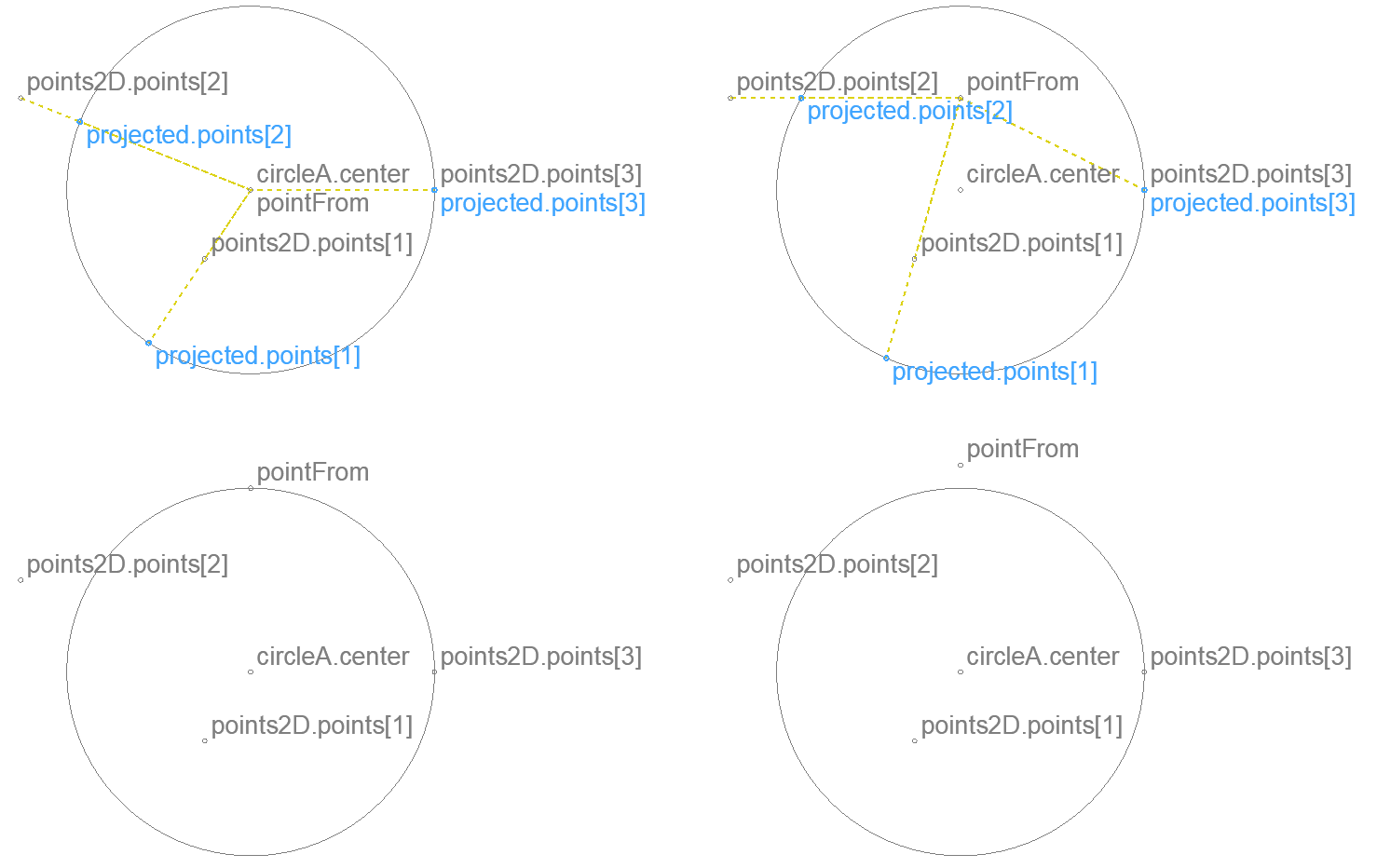## Using BasicGeometry macro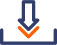Example object for this post

From ARCHICAD 21 a macro called BasicGeometricCalc has been added the ARCHICAD Library. As its name suggests, this macro contains basic geometrical calculations, which can come in handy for GDL 2D and 3D scripting.

With the introduction of DICT in ARCHICAD 23, the parametrization of BasicGeometricCalc has been renewed to use dictionary type parameters and return values in a new macro called BasicGeometry. BasicGeometricCalc is deprecated, only BasicGeometry is updated with new functions. Most of the previously existing functions work the same way with the same geometric data, but some functions of BasicGeometry return more detailed results, some functions have been added, and one function’s return value has changed (see table below).

The macro does geometric calculations. The required method should be given in the macro call with the input data belonging to it. The results of the calculation are given back as returned parameters from the call. It only uses the Master script so it can be called from 2D scripts and 3D scripts as well.

Feel free to save a copy of this macro with a different name, and use it in your own library packages. If any problem or question occurs regarding this macro, please notify us in a comment under this post or in the forum.

### DICT structure documentation conventions

The various functions of the macro use dictionary structures. GDL doesn’t have types to identify dict structures, so we have to document the expected and returned structures. The following format is used:

```name_of_parameter
.key
.subkey1 .subkey2   (type) comment
?   .optional_key
.array[]
.subkey
```

Where parameter type is not written explicitly, it is a dict. Where key type is not written explicitly, it is a real number (floating-point value). Keys that have subkeys – marked with identation – are dict type.

The necessity/existence of optional keys depend on the values of other keys or input values.

The name of a returned parameter can be anything, we only give a suggestion that gives a hint to its meaning.

For example:

```circle
?   .specialPoints[]
.x .y
.center
.x .y
```

This means the following code is valid:

```if haskey(circle.specialPoints) then
for i = 1 to vardim1(circle.specialPoints)
line2 circle.center.x, circle.center.y, circle.specialPoints[i].x, circle.specialPoints[i].y
next i
endif
```

### Geometric concepts used by BasicGeometry

#### accuracy

• Vectors with both coordinates (absolute value) smaller than 0.0001 (1 / 10 mm) are considered null vector.
• Points with both coordinates closer than 0.0001 (1 / 10 mm) are considered the same point.
• Angles (absolute value) smaller than 0.0081° (1 / 10 mm divergence over 1 m) are considered zero.

#### direction vector

A vector that has length 1 (1 meter). It’s length can be changed easily by multiplying all it’s components by the desired length.

#### arc

A circular arc whose central angle’s absolute value is between (excluding) 0° and 360°. The sign of the angle is the same as the direction of rotation from the beginning point to the endpoint (see figure below at polygon).

#### segment

A line between two points that can be either straight or an arc. Defined by it’s two endpoints, type, and central angle for curved segment.

#### edge

A line starting from a point that can be either straight or an arc. Defined similarly to a segment, but omitting the end point. The endpoint is the beginning point of the next edge, thus an edge can’t stand on it’s own.

#### polygon

A collection of edges, without explicitly duplicating the starting point at the end.

The same as a closed polyline.#### polyline

A collection of edges. It can be closed (the same as a polygon) or open: the endpoint can be different than the starting point.

An open polyline is defined by the same number of edges as a closed one, but the last edge is only needed for the endpoint of the polyline, its type is disregarded.

### Functions

The function of the macro can be chosen by the iFunction parameter. The available values of this parameter are referred by named variables in the macro and in this post as well:

 description space iFunction name in BasicGeometry name in BasicGeometricCalc changes in BasicGeometry Direction vector between two points 3D 1 BasicGeometry.DIRECTION_POINTS_3D DIRECTION_POINTS Returns whether input points are the same. Vector mirrored across an axis 2D 2 BasicGeometry.MIRROR_VECTOR_2D MIRROR_VECTOR – Direction vectors of segment end points 2D 3 BasicGeometry.DIRECTION_SEGMENT_2D DIRECTION_SEGMENT Returns whether input points are the same. Intersection of two lines 2D 4 BasicGeometry.INTERSECT_LINE_LINE INTERSECT_LINE_LINE Doesn’t return number of intersections explicitly. Intersection of a circle and a line 2D 5 BasicGeometry.INTERSECT_CIRCLE_LINE_2D INTERSECT_CIRCLE_LINE Doesn’t return number of intersections explicitly. Intersection of a segment and a line 2D 6 BasicGeometry.INTERSECT_SEGMENT_LINE_2D INTERSECT_SEGMENT_LINE Doesn’t return number of intersections explicitly.Returns tangents at intersections. Intersection of a polygon and a line 2D 7 BasicGeometry.INTERSECT_LINE_POLYGON_2D INTERSECT_POLY_LINE Doesn’t return number of intersections explicitly.Returns tangents at intersections. Insert a point onto a segment at given distance from start 2D 8 BasicGeometry.INSERT_POINT_TO_SEGMENT_2D INSERT_POINT_TO_SEGMENT – Segmentation of an arc with given tolerance 2D 9 BasicGeometry.SEGMENT_ARC_2D SEGMENT_ARC – Intersection of two circles 2D 10 BasicGeometry.INTERSECT_CIRCLE_CIRCLE_2D INTERSECT_CIRCLE_CIRCLE – Intersection of a segment and a circle 2D 11 BasicGeometry.INTERSECT_SEGMENT_CIRCLE_2D INTERSECT_SEGMENT_CIRCLE Doesn’t return number of intersections explicitly. Distribute points evenly on polyline 2D 12 BasicGeometry.DISTRIBUTION_POLYLINE_2D DISTRIBUTION_POLY_LINE Doesn’t return number of intersections explicitly. Align Z with vector 3D 13 BasicGeometry.ROT_Z_TO_VECTOR_3D ROTANGLEZ_FOR_NORMVECTOR only available since version AC22 Returned angle is measured at a different location. Project points perpendicularly onto line 2D 14 BasicGeometry.PROJECT_POINTS_LINE_2D not available – Transform points 3D 15 BasicGeometry.LOCAL_POINTS_TO_GLOBAL_3D not available – Inverse-transform points 3D 16 BasicGeometry.GLOBAL_POINTS_TO_LOCAL_3D not available – Arc through three points in order 2D 17 BasicGeometry.ARC_THROUGH_POINTS_2D not available – Project points onto circle from inside 2D 18 BasicGeometry.PROJECT_POINTS_CIRCLE_INNER_2D not available –

#### Direction vector between two points – 3D

Returns the direction vector from `pointFrom `towards `pointTo`, and the distance between them.

##### Input
```pointFrom pointTo
.x .y .z
```

2D calculations can be done by setting z to 0.

##### Result
```direction
.ux .uy .uz
?   .is0		(bool)

distance		(real)
```

Returns a null vector when the two points are closer than 0,00000001 (1 / 100 000 mm). The key direction.is0 only exists in this case, but distance is not necessarily 0.

##### Code snippet
```dict _direction
call "BasicGeometry" parameters iFunction    = BasicGeometry.DIRECTION_POINTS_3D,
PointFrom    = _pointFrom,
PointTo      = _pointTo,
returned_parameters     _direction,
_distance
```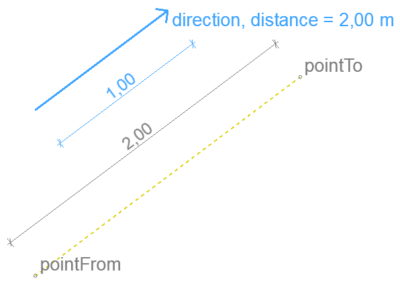#### Vector mirrored across an axis – 2D

Mirrors a direction vector on an axis given by another direction vector.

##### Input
```direction
.ux .uy

mirror
.ux .uy
```

Both input vectors should be direction vectors. This is not checked, but results will be incorrect if their length is not 1.

##### Result
```mirrored
.ux .uy
```

Mirroring a null vector the result is a null vector.

Mirroring on a null vector axis is central inversion, the result will be the opposite of the input.

##### Code snippet
```dict _direction
dict _mirrored
call "BasicGeometry" parameters	iFunction    = BasicGeometry.MIRROR_VECTOR_2D,
mirror	     = _mirror,
direction    = _direction,
returned_parameters    _mirrored
```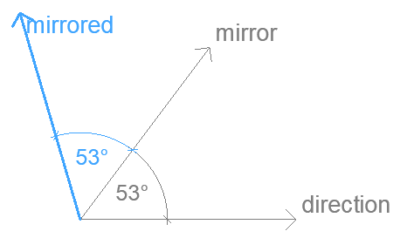#### Direction vectors of segment end points – 2D

Returns the tangents of the segment at the ends, pointing towards the other end.

When the segment is curved, the centerpoint of the arc is returned too.

##### Input
```segment
.begPoint .endPoint
.x .y
.type		(int) 0 - straight, 1 - curved
?   .arcAngle	        Central angle of arc, needed only for curved segment
```

`arcAngle = 0` gives an error, `abs(arcAngle) > 360` gives bad results.

##### Result
```begDirection	        tangential direction at beginning point towards endpoint
.ux .uy
?   .is0		(bool)

endDirection	        tangential direction at endpoint towards beginning point
.ux .uy
?   .is0		(bool)

center
.x .y
```

`center `is (0, 0) for straight segments.

`begDirection `and `endDirection `are null vectors when the segment ends are closer than 0.00000001 (1 / 100 000 mm). The key `is0 `only exists in this case.

##### Code snippet
```dict _begDirection, _endDirection, _center
call "BasicGeometry" parameters	iFunction    = BasicGeometry.DIRECTION_SEGMENT_2D,
segment	     = _segment,
returned_parameters  _begDirection,
_endDirection,
_center
```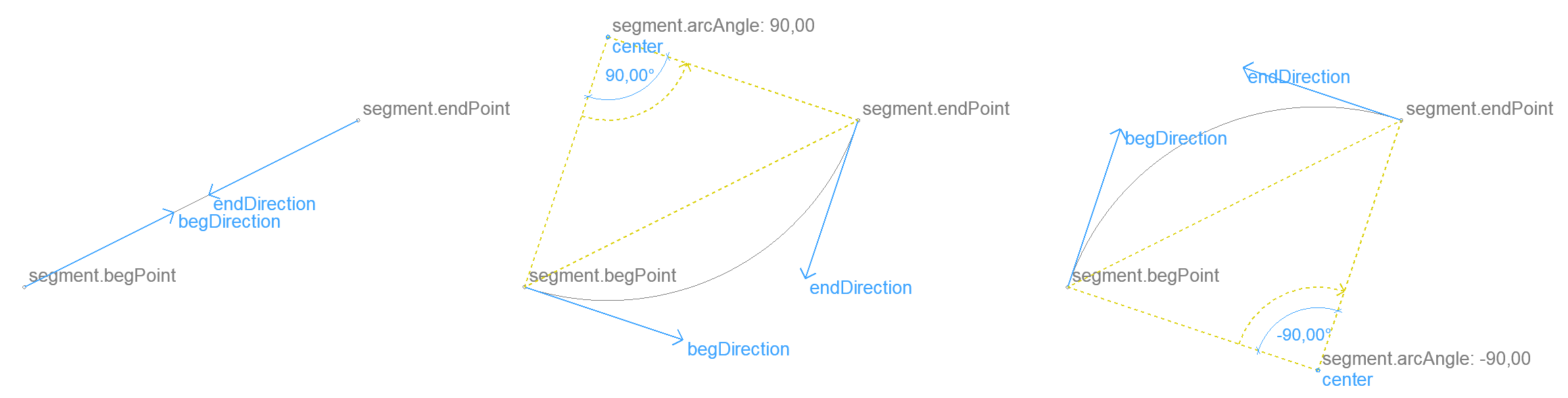#### Intersection of two lines – 2D

Returns the intersection point of two infinite lines.

When the segment is curved, the centerpoint of the arc is returned too.

##### Input
```lineA lineB
.direction
.ux .uy
.point
.x .y
```
##### Result
```intersection
.points[]
.x .y
```

`intersection.points` array size is 1 if there is an intersection, 0 if the lines are parallel or are the same. When the angle between the lines is smaller than 0.0081° (1/10 mm divergence over 1 m) they are considered parallel.

An empty array is returned when any of the input direction vectors is a null vector. The key `is0 `only exists in this case.

##### Code snippet
```dict _intersection
call "BasicGeometry" parameters	iFunction    = BasicGeometry.INTERSECT_LINE_LINE_2D,
lineA	     = _lineA,
lineB	     = _lineB,
returned_parameters  _intersection
```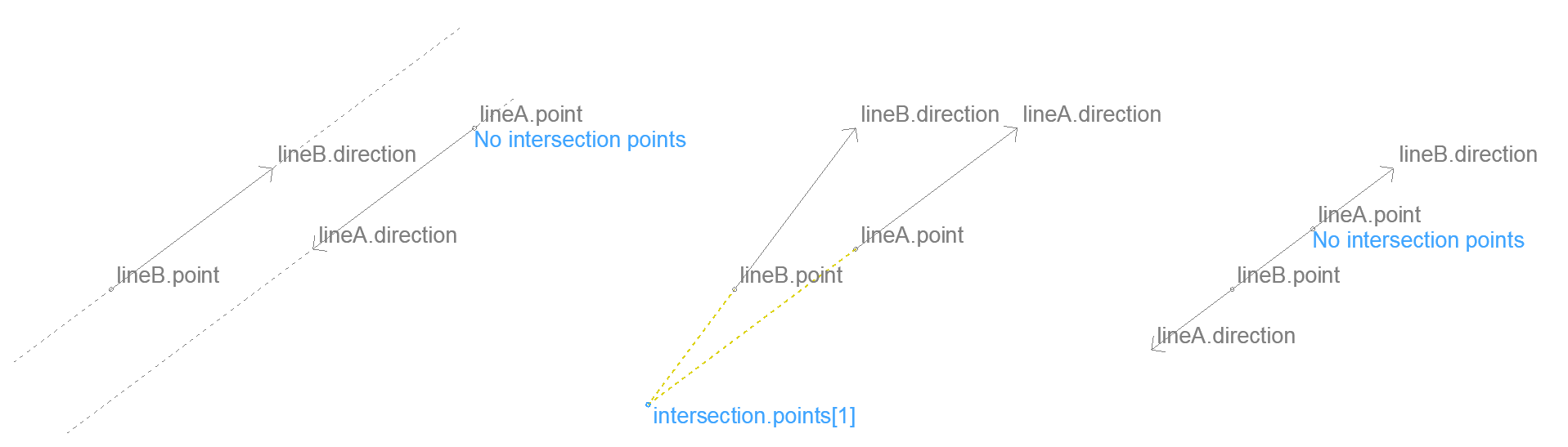#### Intersection of a circle and a line – 2D

Returns the intersection points of a circle and an infinite line.

```lineA
.direction
.ux .uy

circleA
.center
.x .y
```
##### Result
```intersection
.points[]
.x .y
```

`intersection.points` array size is 2 when the line goes through the circle, 1 when it is tangential.

Tangential lines are detected with a precision of 1/10 mm. When `circleA.radius` is smaller than 1/10 mm and the line goes through the circle, only 1 point is returned.

An empty array is returned when there are no intersections.

##### Code snippet
```dict _intersection
call "BasicGeometry" parameters	iFunction    = BasicGeometry.INTERSECT_CIRCLE_LINE_2D,
circleA	     = _circleA,
lineA	     = _lineA,
returned_parameters  _intersection
```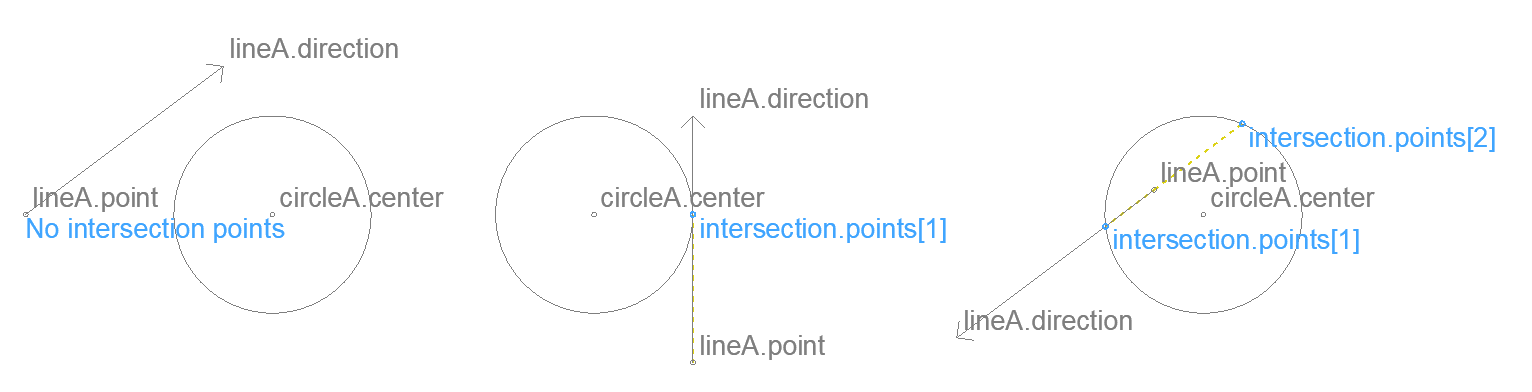#### Intersection of a segment and a line – 2D

Returns the intersection points of a segment and an infinite line.

##### Input
```lineA
.direction
.ux .uy
.point
.x .y

segment
.begPoint .endPoint
.x .y
.type		(int) 0 - straight, 1 - curved
?   .arcAngle	        Central angle of arc, needed only for curved segment
```

`arcAngle = 0` gives an error, `abs(arcAngle) > 360` gives bad results.

##### Result
```intersection
.points[]
.x .y
.tangent	 tangent of segment at intersection
.ux .uy
```

The direction of the tangent of a curved segment is the radius from the arc center to the intersection rotated 90° counterclockwise.

The direction of the tangent of a straight segment is the direction of the segment from the beginning point to the endpoint.

When the segment is straight:

• When the angle between the segment and the line is smaller than 0.0081° (1/10 mm divergence over 1 m) they are considered parallel.

When it is curved:

• When the radius is smaller than 1/10 mm, no intersection is returned even if the line goes through the segment.
• No intersection is returned for a tangential line. Tangential position is detected with a precision of 1/10 mm.

Endpoints are detected with a precision of 1/10 mm.

##### Code snippet
```dict _intersection
call "BasicGeometry" parameters	iFunction    = BasicGeometry.INTERSECT_SEGMENT_LINE_2D,
segment	     = _segment,
lineA	     = _lineA,
returned_parameters  _intersection
```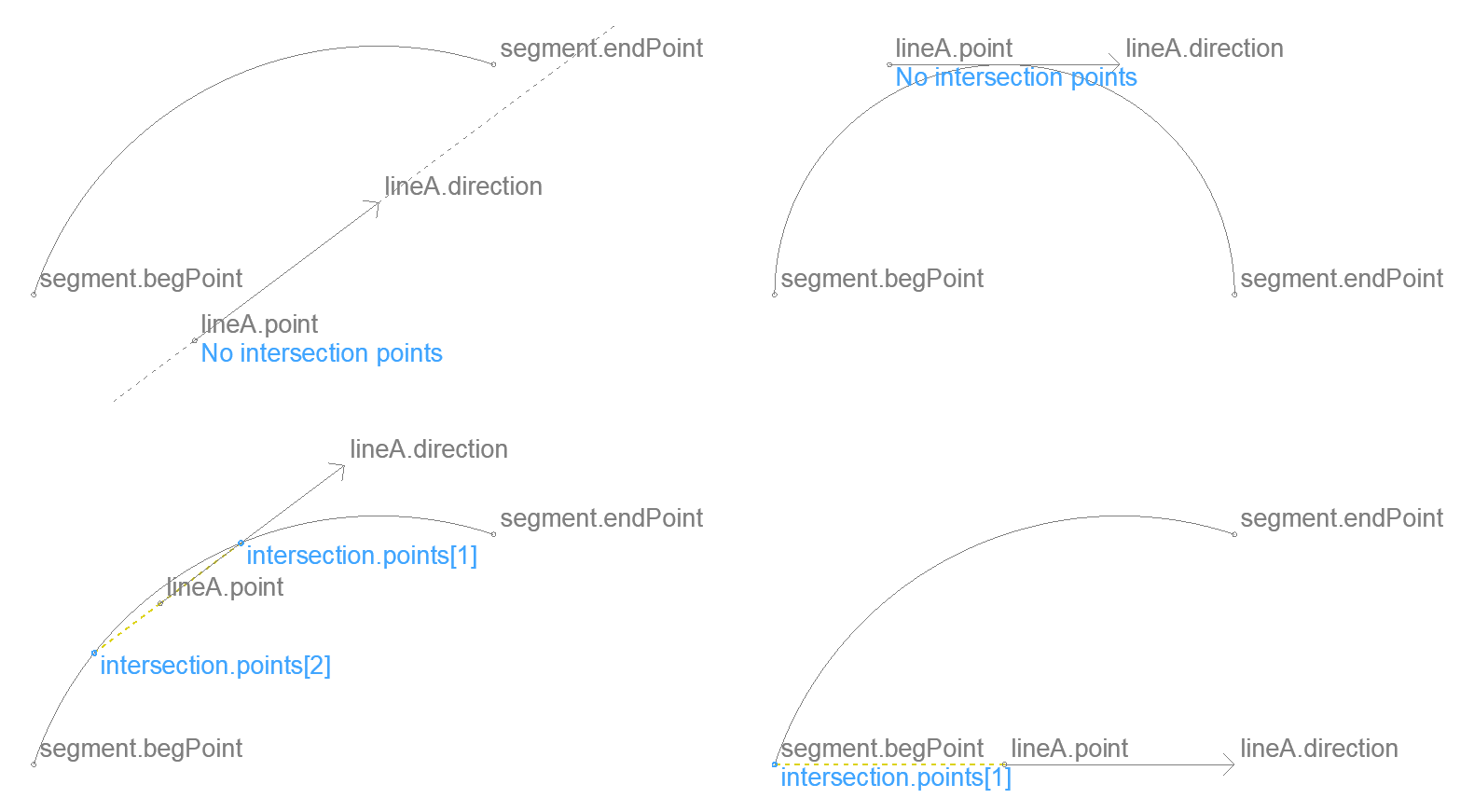#### Intersection of a polygon and a line – 2D

Returns the intersection of a polygon and an infinite line.

##### Input
```polygon
.contour
.edges[]
.begPoint
.x .y
.type	    (int) 0 - straight, 1 - curved
?   .arcAngle	    Central angle of arc, needed only for curved edges
lineA
.direction
.ux .uy
.point
.x .y
```
##### Result
```intersection
.points[]
.x .y
.onSegment	(int) the index of the input edge the intersection is on
.tangent        tangent of edge at intersection
.ux .uy
```

The results are calculated by intersecting the line with each edge as a segment. The same corner cases apply as written at Intersection of a segment and a line.

Intersections at a corner belong to only one edge. When an intersection is close to the beginning of an edge (with 1/10 mm precision), `onSegment `points to the previous edge.

Intersections closer than 1/10 mm are returned only once. This can happen with extremely narrow polygons.

##### Code snippet
```dict _intersection
call "BasicGeometry" parameters	iFunction    = BasicGeometry.INTERSECT_LINE_POLYGON_2D,
polygon      = _polygon,
lineA	     = _lineA,
returned_parameters  _intersection
```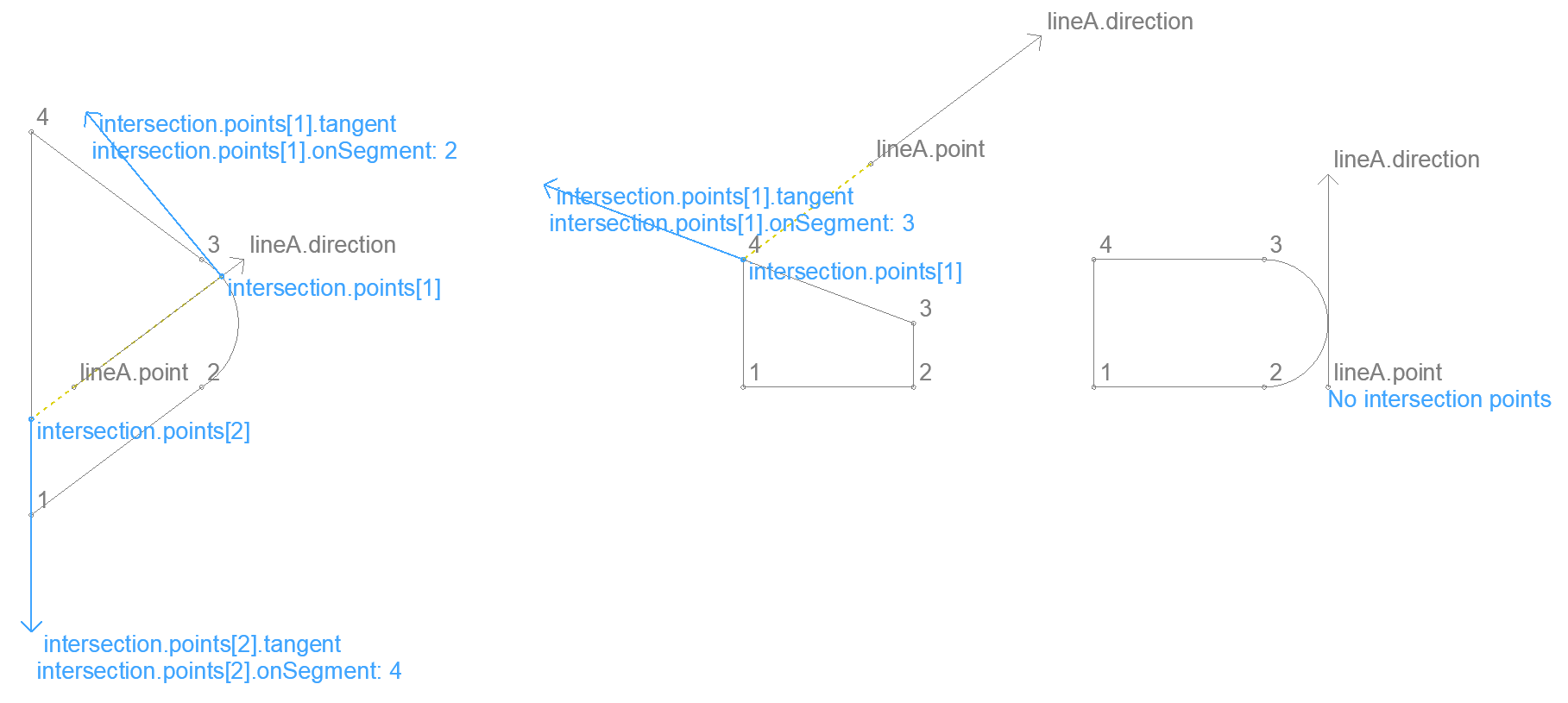#### Insert a point onto a segment at given distance from start – 2D

Returns a point that is at the given distance on a segment. When the segment is curved, distance is measured along the arc.

##### Input
```segment
.begPoint .endPoint
.x .y
.type               (int) 0 - straight, 1 - curved
?   .arcAngle           Central angle of arc, needed only for curved segment

insertionDist		(real) distance
```

`insertionDist `can be negative, or longer than the segment length: the resulting point will be outside the segment (along the same line or circle).

The segment endpoints have to be different, otherwise the results are incorrect.

##### Result
```inserted
.point
.x .y
.angle		arcAngle of the part of the input segment from the beginning point to the inserted point
```

`inserted.angle` can be greater than 360° when `insertionDist `is larger than the length of a full circle along the arc.

`inserted.angle` is 0 when the input segment is straight.

##### Code snippet
```dict _inserted
call "BasicGeometry" parameters	iFunction       = BasicGeometry.INSERT_POINT_TO_SEGMENT_2D,
segment		= _segment,
insertionDist	= insertionDist,
returned_parameters     _inserted
```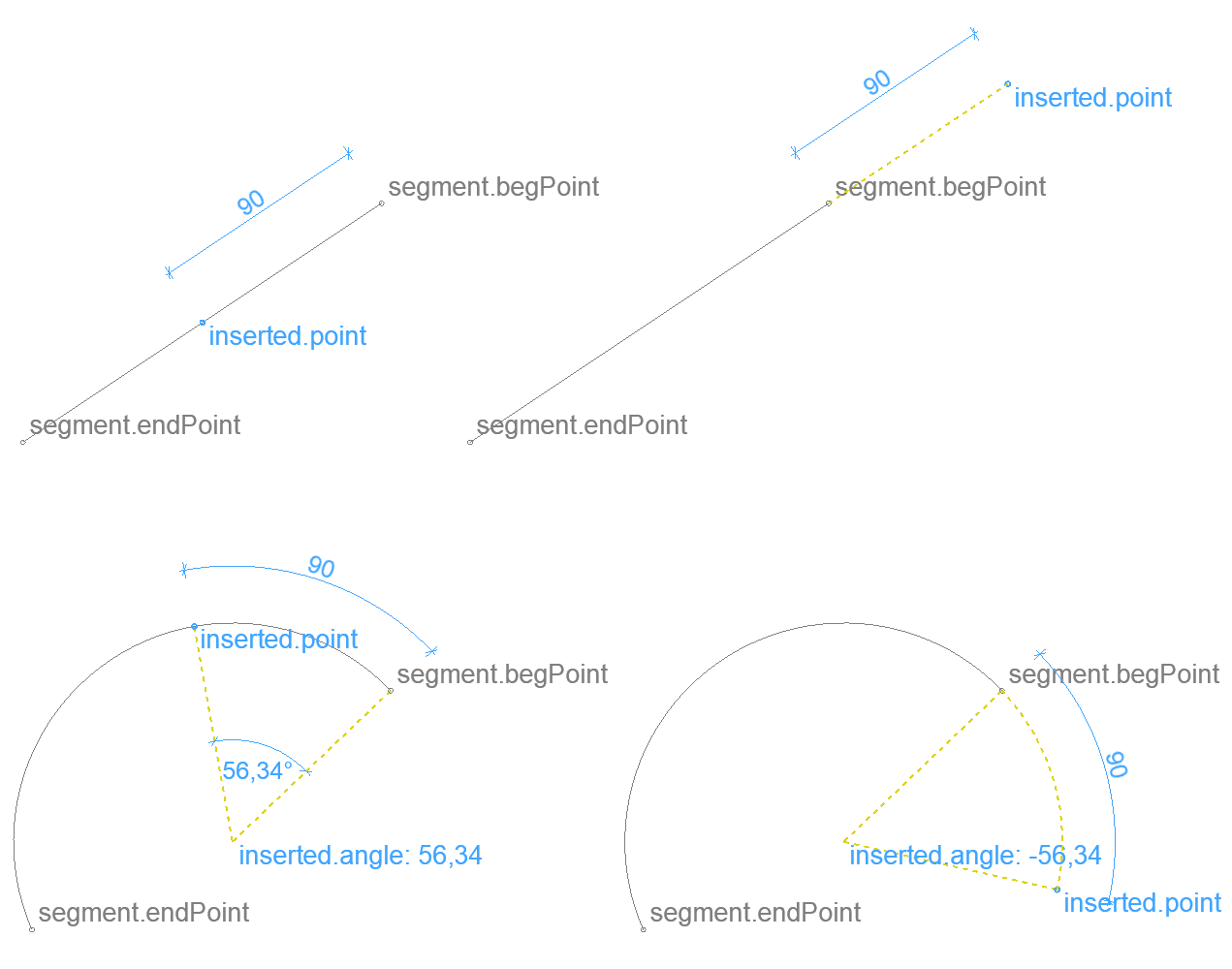#### Segmentation of an arc with given tolerance – 2D

Divides an arc evenly, so that the greatest distance of the chord between two neighboring points and the arc is less than a given length (similar to the TOLER command).

##### Input
```segment
.begPoint .endPoint
.x .y
.type               (int) 0 - straight, 1 - curved
.arcAngle           Central angle of arc

tolerDiff		(real) tolerance
```

When `tolerDiff `is smaller than 1/10 mm, it will be used with the value 1/10 mm. Setting `tolerDiff `larger than the radius of the arc will return the two endpoints.

The segment endpoints have to be different, otherwise the results are bad.

##### Result
```segmented
.points[]
.x .y
```

Altough `segment.type = 0` (straight) is allowed as input, there is no point to call this function on a straight segment. The result will be an empty array.

`inserted.angle` is 0 when the input segment is straight.

##### Code snippet
```dict _segmented
call "BasicGeometry" parameters	iFunction       = BasicGeometry.SEGMENT_ARC_2D,
segment		= _segment,
tolerDiff	= tolerDiff,
returned_parameters     _segmented
```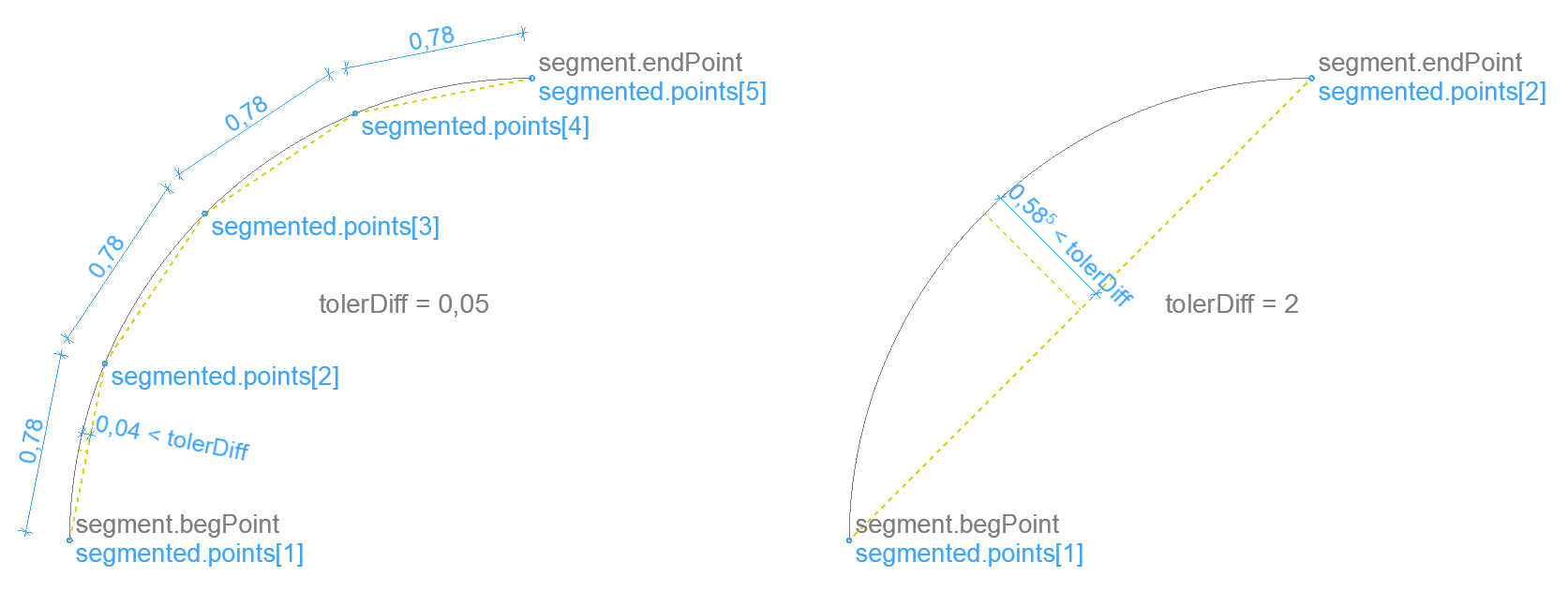#### Intersection of two circles – 2D

Returns the intersection points of two circles.

##### Input
```circleA
.center
.x .y

circleB
.center
.x .y
```
##### Result
```intersection
.count	    (int) number of valid intersections - 0, 1 or 2
.points      always two entries, some of which might be invalid
.x .y
```

Use `intersection.points[]` array elements only up to `intersection.count`, others are invalid.

Circles with equal radius and closer than 1/10 mm are considered the same, no intersection is returned.

Tangential circle positions are calculated with an accuracy of 1/10 mm.

##### Code snippet
```dict _intersection
call "BasicGeometry" parameters	iFunction       = BasicGeometry.INTERSECT_CIRCLE_CIRCLE_2D,
circleA		= _circleA,
circleB		= _circleB,
returned_parameters     _intersection
```#### Intersection of a segment and a circle – 2D

Returns the intersection points of a segment and a circle.

##### Input
```segment
.begPoint .endPoint
.x .y
.type               (int) 0 - straight, 1 - curved
?   .arcAngle           Central angle of arc, needed only for curved segment

circleA
.center
.x .y
```
##### Result
```intersection
.points[]
.x .y
```

When the segment is straight, corner cases written at Intersection of a circle and a line apply.

When the segment is curved, corner cases written at Intersection of two circles apply.

Endpoints, tangential positions are detected with a precision of 1/10 mm.

`intersection.points` array size is the number of found intersections.

##### Code snippet
```dict _intersection
call "BasicGeometry" parameters	iFunction       = BasicGeometry.INTERSECT_SEGMENT_CIRCLE_2D,
segment		= _segment,
circleA		= _circleA,
returned_parameters     _intersection
```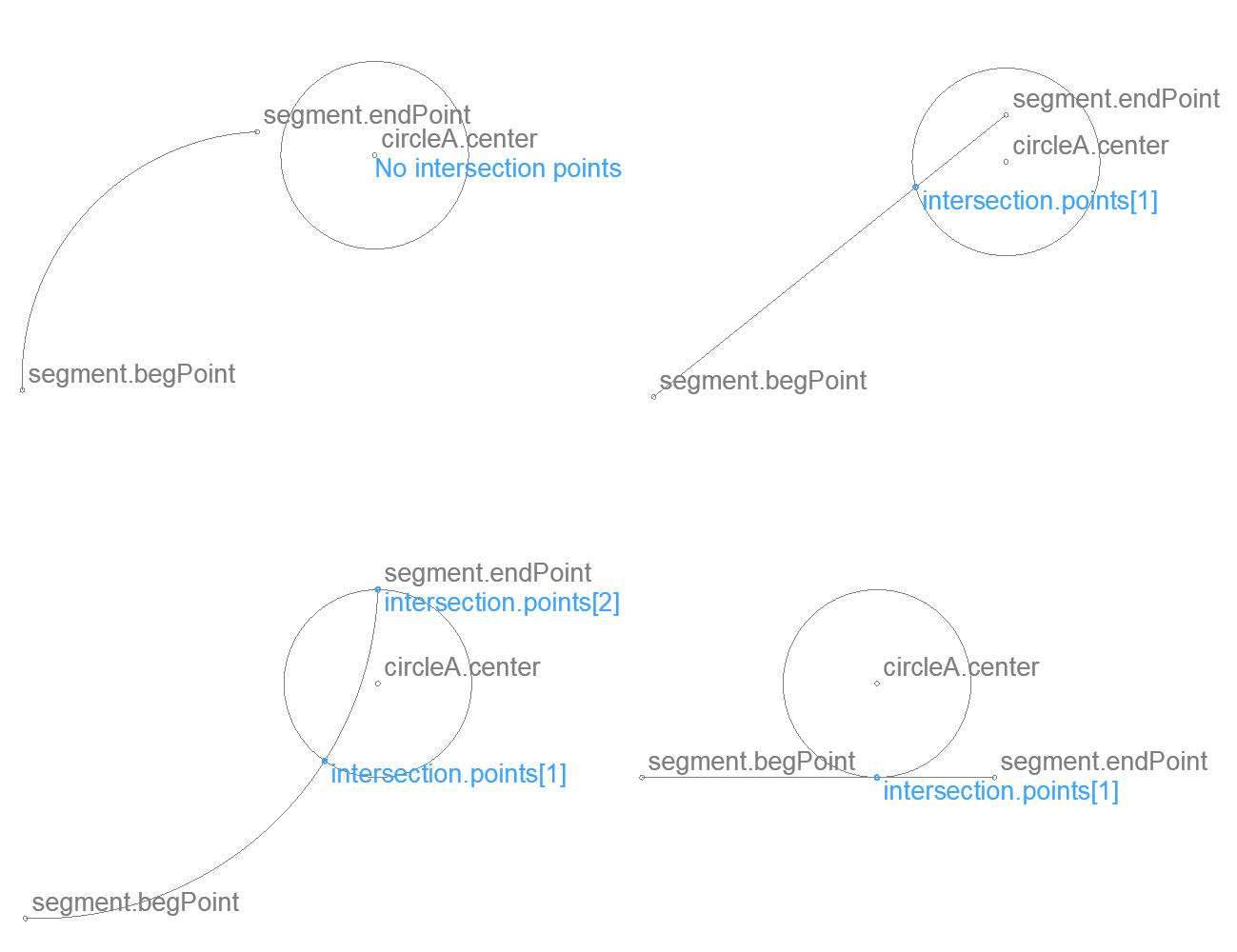#### Distribute points evenly on polyline – 2D

Returns points that are evenly distributed on a polyline. It is possible to define offsets at the two ends of the polyline that are cut off from the distribution.

##### Input
```polyline
.isClosed		    (bool) The polyline is closed (last edge ends at the first edge's begPoint)
.contour
.edges[]
.begPoint
.x .y
.type	    (int) 0 - straight, 1 - curved
?   .arcAngle	    Central angle of arc, needed only for curved edges

distribution
.begOffset
.endOffset
.divisions		    Divide polyline into this # of parts. For example 2 returns 3 points.
```

`distribution.divisions` should be greater than 0. For practical use it should be an integer.

With `distribution.divisions = 1` offsets from both ends of the polyline are returned.

The `distribution` offsets are used with their absolute value. When the sum of the two offsets is longer than the polyline, no points are returned.

##### Result
```segmented
.points[]
.x .y
.onSegment	    (int) Index of the input polyline edge on which the point is.
.crossing	    Direction vector perpendicular to the polyline at the point.
.ux .uy
```

Points near a corner belong to the edge ending there (with 1/10 mm precision).

`crossing `direction is always pointing to the right side of the polyline (looking in the direction of the polyline).

##### Code snippet
```dict _segmented
call "BasicGeometry" parameters	iFunction       = BasicGeometry.DISTRIBUTION_POLYLINE_2D,
polyline	= _polyline,
distribution	= _distribution,
returned_parameters     _segmented
```#### Align Z with vector – 3D

Returns the necessary rotation axis and angle that rotates the Z axis to be parallel with the input vector.

##### Input
```vector
.dx .dy .dz
```

The input vector’s length should be greater than 0.

##### Result
```rotation
.axis			direction vector of rotation axis
.dx .dy .dz
.angle			necessary rotation around .axis, degrees
```
##### Code snippet
```dict _rotation
call "BasicGeometry" parameters	iFunction       = BasicGeometry.ROT_Z_TO_VECTOR_3D,
vector	        = _cutPlaneNormal,
returned_parameters     _rotation
```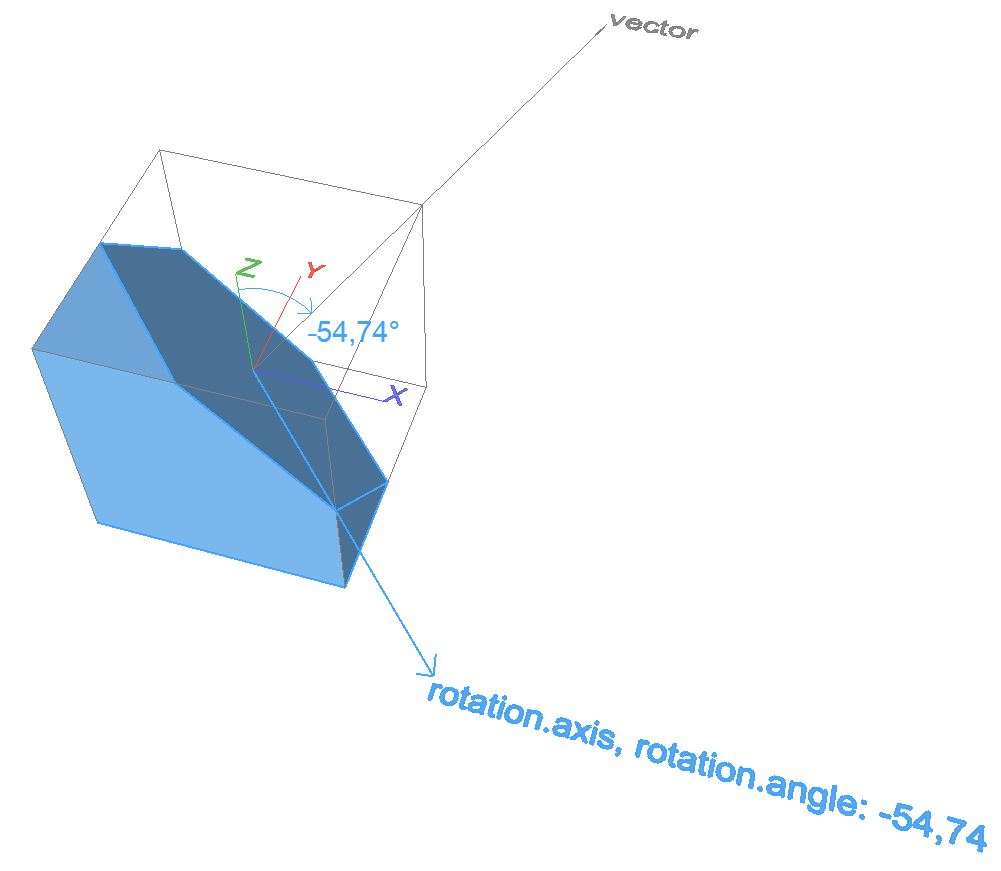#### Project points onto line – 2D

Projects points perpendicularly onto a line.

##### Input
```lineA
.point			point on line
.x .y
.direction		        direction vector parallel with line
.ux .uy

points2D
.points[]
.x .y
```

`points2D.points[]` array size has to be at least 1.

##### Result
```projected
.points[]
.x .y
```

`projected.points[]` array has the same number of elements as input `points2D.points[]` array.

##### Code snippet
```dict _projected
call "BasicGeometry" parameters	iFunction       = BasicGeometry.PROJECT_POINTS_LINE_2D,
points2D	= _points2D,
lineA		= _lineA,
returned_parameters     _projected
```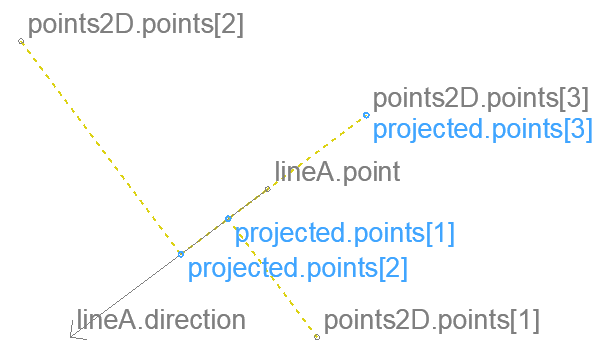#### Transform points – 3D

Returns the coordinates of local points expressed in a global coordinate system.

Can be used to calculate global coordinates of points in a local coordinate system described by an XFORM-style transformation matrix (global coordinates of points placed in transformed coordinate system).

Can be used to transform points with an XFORM-style transformation matrix, returning the coordinates after the transformation.

##### Input
```points3D
.points[]
.x .y .z

transformation
.origin		origin after the transformation
.x .y .z
.XAxis		vector of transformed X axis, relative to transformed origin
.dx .dy .dz
.YAxis		vector of transformed Y axis, relative to transformed origin
.dx .dy .dz
.ZAxis		vector of transformed Z axis, relative to transformed origin
.dx .dy .dz
```

`points3D `must contain at least an empty `points[]` array.

##### Result
```global
.points[]
.x .y .z
```

`global.points[]` array has the same number of elements as input `points3D.points[]` array.

##### Code snippet
```dict _global
call "BasicGeometry" parameters	iFunction       = BasicGeometry.LOCAL_POINTS_TO_GLOBAL_3D,
transformation  = _transformation,
points3D        = _points3D,
returned_parameters     _global
```

There are two ways to visualize the operation:

#### Inverse-transform points – 3D

Returns the coordinates of global points expressed in a local coordinate system.

Can be used to calculate local coordinates of points in the global coordinate system. The local coordinate system is described by an XFORM-style transformation matrix (local coordinates of points placed in the global coordinate system).

Can be used to transform points with the inverse of a transformation matrix, returning the coordinates before the transformation.

##### Input
```points3D
.points[]
.x .y .z

transformation
.origin		origin after the transformation
.x .y .z
.XAxis		vector of transformed X axis, relative to transformed origin
.dx .dy .dz
.YAxis		vector of transformed Y axis, relative to transformed origin
.dx .dy .dz
.ZAxis		vector of transformed Z axis, relative to transformed origin
.dx .dy .dz
```

`points3D `must contain at least an empty `points[]` array.

##### Result
```local
.points[]
.x .y .z
```

`local.points[]` array has the same number of elements as input `points3D.points[]` array.

##### Code snippet
```dict _local
call "BasicGeometry" parameters	iFunction       = BasicGeometry.GLOBAL_POINTS_TO_LOCAL_3D,
transformation  = _transformation,
points3D        = _points3D,
returned_parameters     _local
```

There are two ways to visualize the operation:

#### Arc through three points in order – 2D

Returns an arc that goes through the input points in order. Returns the polar angle of the input points relative to the X-axis.

##### Input
```points2D
.points		three points in order: beginning, intermediate, end
.x .y
```
##### Result
```arc2D
.exists		(bool) Is there a valid solution?
.center		center of circle
.x .y
.begAngle		polar angle of beginning point
.midAngle		polar angle of intermediate point
.endAngle		polar angle of end point
```

A valid solution doesn’t exist when any two of the input points are the same, or all three of them lie on the same line.

The returned polar angles are ordered ( `sgn(begAngle - midAngle) = sgn(midAngle - endAngle)` ). Their absolute value can be larger than 360°, their value can be lower than 0°. The absolute value of the difference between two angles is always less than 360°. This means the relative angle between any two points can be easily passed to GDL polygon commands using status code 4000.

##### Code snippet
```dict _arc
call "BasicGeometry" parameters	iFunction       = BasicGeometry.ARC_THROUGH_POINTS_2D,
points2D        = _points2D,
returned_parameters     _arc
```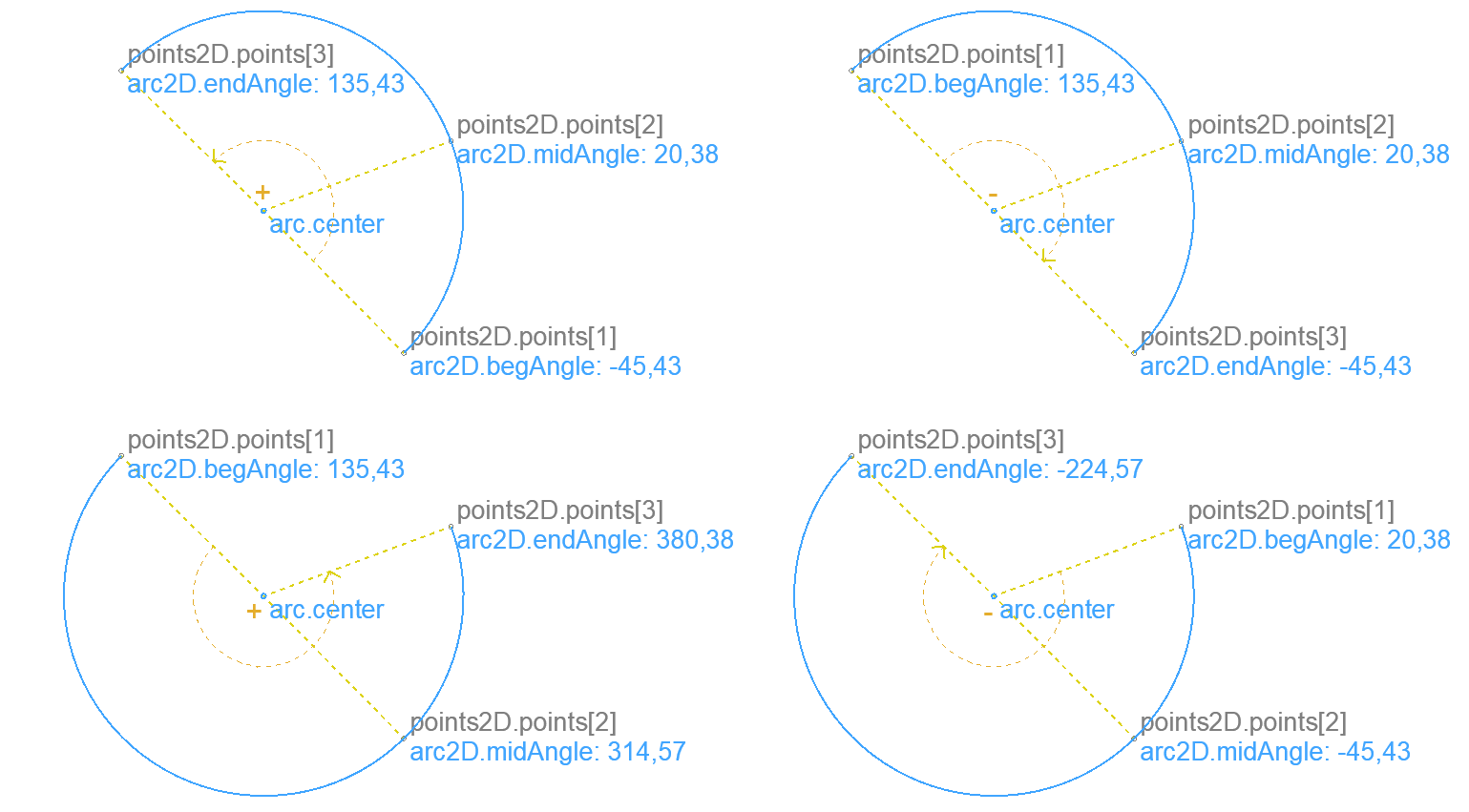#### Project points onto circle from inside – 2D

Project points onto circle from a point inside the circle.

##### Input
```points2D
.points[]
.x .y

pointFrom
.x .y

circleA
.center
.x .y
```

`pointFrom `should be a point inside the circle.

`points2D.points[]` can be anywhere in the plane except `pointFrom`.

##### Result
```projected
.points[]
.x .y
```

Each input point will have exactly one corresponding projected point. Input points that are the same as `pointFrom `are returned unchanged.

An empty `projected.points[]` array is returned when `pointFrom `wasn’t inside the circle.

##### Code snippet
```dict _projected
call "BasicGeometry" parameters	iFunction       = BasicGeometry.PROJECT_POINTS_CIRCLE_INNER_2D,
points2D        = _points2D,
pointFrom       = _pointFrom,
circleA         = _circleA,
returned_parameters     _projected
```